IMO Shortlist 2011 problem N5

Kvaliteta:
Avg: 0,0
Težina:
Avg: 8,0
Let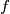$f$ be a function from the set of integers to the set of positive integers. Suppose that, for any two integers$m$ and$n$, the difference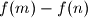$f(m) - f(n)$ is divisible by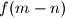$f(m- n)$. Prove that, for all integers$m$ and$n$ with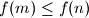$f(m) \leq f(n)$, the number$f(n)$ is divisible by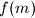$f(m)$.

Proposed by Mahyar Sefidgaran, Iran
Izvor: Međunarodna matematička olimpijada, shortlist 2011